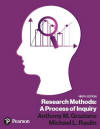﻿ Statistical Concepts TutorialGraziano & Raulin
Research Methods (9th edition)

## Statistical Concepts

The Graziano and Raulin text provides brief overviews of statistical concepts and when to use statistical tests, and the Student Resource Website provides extensive explanations of how to compute various statistics, but neither of these are sufficient to understand the concepts behind statistics.

This section of the Student Resource Website contains descriptions of practical processes, such as statistical notation, and explanations of statistical concepts, such as probability. By itself, this section will not replace a good course in statistics, but it can provide the motivated student with a conceptual understanding of statistical procedures that is sufficient to employ statistical procedures correctly in the analysis of research.

The table below provides quick links to the various sections of the Statistical Concepts tutorial. Below the table is a description of what is covered in each of those sections. We recommend that you cover the topics in the order that they are presented, because many of the later topics build on concepts discussed in earlier sections.

In the table, the topics in larger type are the section titles. The topics in smaller type are specific topics, which are conceptually equivalent to chapters in a textbook. Each section is linked to the next section with a button at the bottom of the page, so you can read this material in much the same with that you would read a textbook. Finally, there is a separate index at the bottom of the table to help you find specific information on statistical concepts quickly. The items in this index are also included in the index for the Student Resource Website, although that index covers everything that is included on the Website.

### Basic Inferential Statistics

Nature of Statistics      One-Sample t-test
Organizing and Graphing Data      Independent Samples t-test
Statistical Notation

### Descriptive Statistics

Basic One-Way ANOVA
Central Tendency      Probing Techniques
Variability
Relative Scores      Basic Two-Way ANOVA
Measures of Relationship

### Logic of Inferential Statistics

Other Nonparametric Statistics
Probability

### Conclusions

Sampling Distributions

## Description of Each Section

### Understanding Statistics

• Nature of Statistics. This section describes the purpose of statistics and introduces you to some basic terminology.

• Organizing and Graphing Data. This section describes how data are collected and how they are organized in tables and graphs.

• Statistical Notation. This section introduces the concept of statistical notation, which is used in the various formulas that will be introduced.

### Descriptive Statistics

• Central Tendency. This section covers the various measures of central tendency.

• Variability. This section covers the various measures of variability.

• Relative Scores. This section covers the concept of relative scores and the various types of relative scores that are available.

• Measures of Relationship. This section introduces the concept of relationships and the various measures of relationship.

• Other Descriptive Statistics. This section defined skewness and kurtosis.

### Logic of Inferential Statistics

• Probability. This section introduces the concept of probability and relates that concept to distributions, especially the normal distribution.

• Sampling Distributions. This section introduces the concept of sampling distributions and how they are affected by such variables as sample size.

• Inferential Statistics. This section introduces the concept of inferential statistics and the logic behind such statistics. It connects the concepts of probability and sampling distributions to the process for drawing inferences about populations from sample data.

### Basic Inferential Statistics

• One-Sample t-test. This section introduces the concept of null hypothesis testing using the one-sample t-test.

• Independent Samples t-test. This section introduces the concept of comparing two independent samples using the t-test.

• Correlated Samples t-test. This section introduces the t-test for correlated samples and the logic behind it.

• Basic One-Way ANOVA. This section introduces the logic of analysis of variance and the use of analysis of variance to evaluate mean differences in more than two independent groups.

• Probing Techniques. This section covers planned comparisons and post hoc tests.

• Repeated Measures ANOVA. This section introduces the repeated measures ANOVA, linking that procedure to the t-test for correlated groups.

• Basic Two-Way ANOVA. This section introduces the ANOVA for a completely randomized two-factor design.

### Nonparametric Statistics

• Chi Square for Categorical Data. This section introduces the Chi Square distribution and shows how to use the distribution to test how well data fit a given hypothesis and how to use the distribution to compare two of more groups.

• Other Nonparametric Techniques. This section catalogs several other frequently-used nonparametric techniques, without going into detail about how they are computed.